Engineering Jobs   »   QUIZ : CIVIL ENGINEERING (08-12-2020)

# QUIZ : CIVIL ENGINEERING (08-12-2020)

Quiz: Civil Engineering
Exam: UPPSC
Topic: STEEL STRUCTURE

Each question carries 1 mark
Negative marking: 1/3 mark
Time: 15 Minutes

Q1. If ‘M’ is maximum moment in the plate girder, ‘P’ is allowable bending stress and ‘t_w, is the thickness of web, Economical depth for a plate girder is given by-
(a) √((M\/P)/t_w )
(b) (1.2√(M\/P))/t_w
(c) (1.1√(M\/P))/t_w
(d) (1.3√(M\/P))/t_w

Q2. A steel beam is carrying just its self load. it needs to be so supported on two columns (span length, L) with equal overhangs (Y) on both the sides such that the bending moment at the mid-span is zero. The ratio of L/Y should be:
(a) 0.25
(b) 0.5
(c) 1.00
(d) 2.00

Q3. The depth of foundation is usually calculated from?
(a) De Almberts formula
(b) Rankine’s formula
(c) Newton formula
(d) Gutters formula

Q4. Find Euler’s crippling load for a steel column of effective length 2 m. take E 2×10^5 N/mm² and cross section as 2cm (wide) ×4cm (deep) rectangular.
(a) 28.32 kN
(b) 13.16 kN
(c) 52.64 kN
(d) 26.32 kN

Q5. A structural member subject to compressive force in a direction parallel to its longitudinal axis is called
(a) Column
(b) Post
(c) Stanchion
(d) Any one of above

Q6. What should be the minimum thickness of the slab base of size 400mm×400mm below a column ISHB 300(A=7485mm², b = 250mm) carrying an axial load of 200kN, if the permissible bending stress is 185 MPa?
(a) 6mm
(b) 8mm
(c) 10mm
(d) 12mm

Q7. Refer Rankine’s empirical correlation for buckling load of an intermediate column. The value of constant and will be least for a column made of ___________:
(a) Cast iron
(b) Wrought iron
(c) Mile steel
(d) Timber

Q8. The maximum spacing of vertical stiffeners in plate girder is limited to
(a) 0.33d
(b) 0.5d
(c) d
(d) 1.5d

Q9. Maximum bending moment in roof purlins is taken as
(a) WL/10
(b) WL/20
(c) WL/40
(d) WL/50

Q10. The line of intersection of the surfaces of a sloping roof forming an external angle exceeding 180° is
(a) ridge
(b) hip
(c) valley
(d) None of these

SOLUTION

S1. Ans.(c)
Sol. in general, the depth of plate girder is kept as 1/10 to 1/12 of span.
Economical depth of plate girder→
▭(D=(1.1√M)/(P×tw))
Where,
M = maximum bending moment
tw = thickness of web
P = Allowable bending stress
S2. Ans.(d)
Sol. When a steel beam is carrying just its self load and it needs to be supported on two column (span length, L) with equal overhangs (Y) on both the sides such that the bending moment at the mid span is zero, then the ratio of L/y should be 2.00.

S3. Ans.(b)
Sol. the depth of foundation is usually calculated from Rankine formula and it is given by –
▭(D=P/W ((1-Sinθ)/(1+Sinθ))^2 )
D = Depth of foundation
P = gross bearing capacity
W= density of soil
θ = angle of response of soil

S4. Ans.(c)
Sol. Given,
Effective length of column (l) = 2m = 2000mm
Cross -section area of rectangular section = (2 × 4) cm² = 800 m²
Moment of inertia of rectangular section (Ixx)
=(bh^3)/12
=(20×(40)^3)/12
=106666.66 mm^4
Euler’s crippling load (Pcr) = (π^2 EI)/l^2
=((3.141)^2×2×10^5×106666.66)/(4×10^6 )
=(9.85×106666.66×100)/40
=52618.30 N or 52.63 kN
S5. Ans.(c)
Sol. stanchion is a structural member subjected to compression force in a direction parallel to its longitudinal axis.
S6. Ans.(c)
Sol.

Given, Size of slab base = 400mm × 400 mm
Axial load (Pu) = 200 kN
Permissible bending stress (σ_br ) = 185 MPa
A = 7485 mm², b = 250 mm.
W=P/Area=(200×10^3)/(400×400)=1.25 N\/mm^2
a=(400-250)/2=75mm
b=(400-300)/2=50 mm
According to IS 800 – 2007
Thickness of plate (t) = √(3w(a^2-0.3b^2 )/σ_bs )
√((3×1.25(75^2-0.3×50^2 ))/185)
=9.94≈10 mm.

S7. Ans.(b)
Sol.
Material Rankine Constant (a=f_c/(π^2 E))
Cast iron 1/1600
Mild steel 1/7500
Wrought iron 1/9000
Wood 1/3000
S8. Ans.(d)
Sol. in plate girders, vertical stiffeners are required when the ratio of clear depth of web (d) to web thickness greater than 85. its normal distance kept between 0.33 d to 1.5 d.
S9. Ans.(a)
Sol. the maximum bending moment in roof purlins is taken as (‘WL^’)/10 or (‘wL^(2^’ ))/10
W= total load (KN) = wL

S10. Ans.(b)
Sol. the line of intersection of the surfaces of a slopping roof forming an external angle exceeding 180° is hip.

Sharing is caring!

Thank You, Your details have been submitted we will get back to you.
•How to Prepare for SSC JE 2022 General A...
•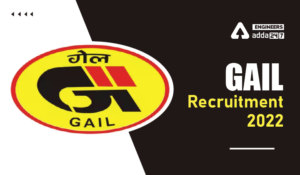GAIL Recruitment 2022, GAIL 282 Non Exec...
•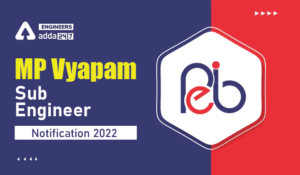MP Vyapam Sub Engineer Notification 2022...
•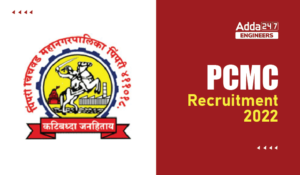PCMC Recruitment 2022, Check here the de...
•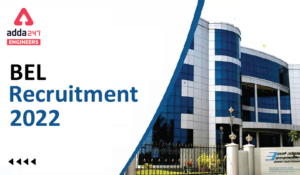BEL Recruitment 2022, Check here For 13 ...
•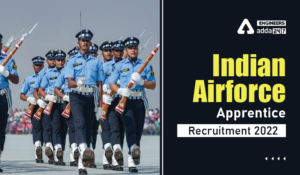Indian Air Force Apprentice Recruitment ...